Warning: unlink(/var/www/html/images/tmp/7ee528f7a3b5c3fc62b41736f86b2873.asy): No such file or directory in /var/www/html/extensions/Asymptote.php on line 109

Warning: unlink(/var/www/html/images/tmp/ltx-7ee528f7a3b5c3fc62b41736f86b2873.aux): No such file or directory in /var/www/html/extensions/Asymptote.php on line 113

Warning: unlink(/var/www/html/images/tmp/ltx-7ee528f7a3b5c3fc62b41736f86b2873.log): No such file or directory in /var/www/html/extensions/Asymptote.php on line 114

Warning: unlink(/var/www/html/images/tmp/7ee528f7a3b5c3fc62b41736f86b2873.eps): No such file or directory in /var/www/html/extensions/Asymptote.php on line 115

Warning: unlink(/var/www/html/images/tmp/7ee528f7a3b5c3fc62b41736f86b2873.log): No such file or directory in /var/www/html/extensions/Asymptote.php on line 116
Difference between revisions of "User:Nikita2/sandbox" - Encyclopedia of Mathematics

# Difference between revisions of "User:Nikita2/sandbox"

A mapping $\varphi:D\to D'$ possesses Luzin's $\mathcal N$-property if the image of every set of measure zero is a set of measure zero. A mapping $\varphi$ possesses Luzin's $\mathcal N{}^{-1}$-property if the preimage of every set of measure zero is a set of measure zero.

Briefly \begin{equation*} \mathcal N\text{-property:}\quad \Sigma\subset D, |\Sigma| = 0 \Rightarrow |\varphi(\Sigma)|=0, \end{equation*} \begin{equation*} \mathcal N{}^{-1}\text{-property:} \quad M \subset D, |M| = 0 \Rightarrow |\varphi^{-1}(M)|=0. \end{equation*}

## Contents

#### $\mathcal N$-property of a function $f$, continuous on an interval $[a,b]$

For any set $E\subset[a,b]$ of measure zero ($|E|=0$), the image of this set, $f(E)$, also has measure zero. It was introduced by N.N. Luzin in 1915 (see ). The following assertions hold.

1. A function $f\not\equiv \operatorname{const}$ on $[a,b]$ such that $f'(x)=0$ almost-everywhere on $[a,b]$ (see for example Cantor ternary function) does not have the Luzin $\mathcal N$-property.
1. Ifdoes not have the Luzin-property, then onthere is a perfect setof measure zero such that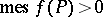.
1. An absolutely continuous function has the Luzin-property.
1. Ifhas the Luzin-property and has bounded variation on(as well as being continuous on), thenis absolutely continuous on(the Banach–Zaretskii theorem).

5) Ifdoes not decrease onand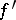is finite on, thenhas the Luzin-property.

6) In order that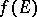be measurable for every measurable set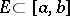it is necessary and sufficient thathave the Luzin-property on.

7) A functionthat has the Luzin-property has a derivativeon the set for which any non-empty portion of it has positive measure.

8) For any perfect nowhere-dense set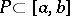there is a functionhaving the Luzin-property onand such thatdoes not exist at any point of.

The concept of Luzin's-property can be generalized to functions of several variables and functions of a more general nature, defined on measure spaces.

How to Cite This Entry:
Nikita2/sandbox. Encyclopedia of Mathematics. URL: http://encyclopediaofmath.org/index.php?title=Nikita2/sandbox&oldid=29210